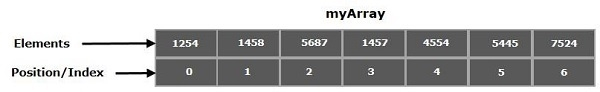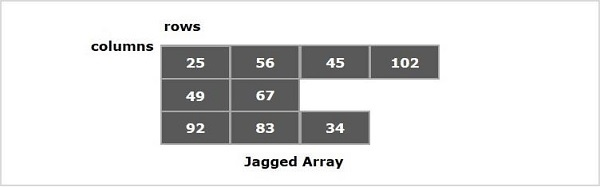# What are jagged arrays and explain with an example in Java?

Arrays are the containers that store multiple variables of the same datatype. These are of fixed size which is determined at the time of creation. Each element in an array is positioned by a number starting from 0.You can access the elements of an array using name and position as −

System.out.println(myArray);
//Which is 1457

In Java, arrays are treated as referenced types you can create an array using the new keyword similar to objects and populate it using the indices, while creating array in this way, you must specify its size as −

int myArray[] = new int;

You can also directly assign values within flower braces separating them with commas (,) as −

int myArray = {1254, 1458, 5687, 1457, 4554, 5445, 7524};

## Jagged arrays

You can have arrays with in an array these are known as multi-dimensional arrays.

2D array − A two dimensional array in Java is represented as an array of one dimensional arrays of the same type. Mostly, it is used to represent a table of values with rows and columns −

Int[][] myArray = {{10, 20, 30}, {11, 21, 31}, {12, 22, 32} }

Multi-dimensional arrays in Java with variable number of columns is known as jagged array. For example, we can create a 2D array where first array is of 3 elements, and is of 4 elements.## Example

Following Java program defines, populates a Jagged array and prints its contents.

import java.util.Arrays;
public class JaggedArraysExample {
public static void main(String args[]){
//Array with 3 rows and variable columns
int[][] myArray = new int[];
//0th row with 2 columns
myArray = new int;
//1st row with 5 columns
myArray = new int;
//2nd row with 4 columns
myArray = new int;
//Populating 0th row
myArray = 21;
myArray = 22;
//Populating 1st row
myArray = 24;
myArray = 25;
myArray = 26;
myArray = 27;
myArray = 28;
//Populating 2nd row
myArray = 29;
myArray = 30;
myArray = 31;
myArray = 32;
for(int i=0; i<myArray.length; i++ ){
System.out.println(Arrays.toString(myArray[i]));
}
}
}

## Output

[21, 22]
[24, 25, 26, 27, 28]
[29, 30, 31, 32]